[BACK]Computer Modeling in Engineering & SciencesDOI: 10.32604/cmes.2021.015736

ARTICLE

Mining Bytecode Features of Smart Contracts to Detect Ponzi Scheme on Blockchain

1Henan Key Laboratory of Big Data Analysis and Processing, Henan University, Kaifeng, 47500, China
2Institute of Data and Knowledge Engineering, Henan University, Kaifeng, 47500, China
3School of Computer and Information Engineering, Henan University, Kaifeng, 47500, China
*Corresponding Author: Lei Zhang. Email: zhanglei@henu.edu.cn
Received: 09 January 2021; Accepted: 24 February 2021

Abstract: The emergence of smart contracts has increased the attention of industry and academia to blockchain technology, which is tamper-proofing, decentralized, autonomous, and enables decentralized applications to operate in untrustworthy environments. However, these features of this technology are also easily exploited by unscrupulous individuals, a typical example of which is the Ponzi scheme in Ethereum. The negative effect of unscrupulous individuals writing Ponzi scheme-type smart contracts in Ethereum and then using these contracts to scam large amounts of money has been significant. To solve this problem, we propose a detection model for detecting Ponzi schemes in smart contracts using bytecode. In this model, our innovation is shown in two aspects: We first propose to use two bytes as one characteristic, which can quickly transform the bytecode into a high-dimensional matrix, and this matrix contains all the implied characteristics in the bytecode. Then, We innovatively transformed the Ponzi schemes detection into an anomaly detection problem. Finally, an anomaly detection algorithm is used to identify Ponzi schemes in smart contracts. Experimental results show that the proposed detection model can greatly improve the accuracy of the detection of the Ponzi scheme contracts. Moreover, the F1-score of this model can reach 0.88, which is far better than those of other traditional detection models.

Keywords: Ponzi scheme; blockchain security; smart contracts; anomaly detection

1  Introduction

Blockchain technology originated from Nakamoto  invention of Bitcoin technology, which is a distributed accounting technology that builds trust and achieves node consensus in a peer-to-peer network. On the one hand, the consensus mechanism is used to solve the information consistency problem in the untrusted environment to guarantee that all nodes have the same transaction information. On the other hand, cryptographic techniques such as digital signatures and hashing algorithms are used to guarantee the security of the distributed network. The use of these technologies has made blockchain decentralized, tamper-proof, open, and transparent. The emergence of smart contracts gives blockchain the ability to truly change the world . In 1995, Nick Szabo already introduced the concept of a smart contract ; “a smart contract is a set of digitally defined promises, including an agreement on which the contract participants can execute those promises.” In blockchain terminology, a smart contract is a piece of programming code that runs on the blockchain system. By writing the execution conditions and steps of the contract into the blockchain system, the contract can be triggered to execute automatically when a specific event occurs (unless otherwise specified, all smart contracts mentioned below refer specifically to smart contracts in the blockchain) .

Smart contracts inherit the characteristics of blockchain, which also has the characteristics of decentralization, tamper-proofing, openness, and transparency; these properties make smart contracts have a broad application prospect. Ethernet is one of the world’s leading open-source blockchain platforms, it implements support for smart contracts using the Ethernet virtual machine (EVM), and allows developers to write smart contracts using Turing-complete languages such as Solidity . According to Dune Analytics , the total number of smart contracts published on the Ethereum blockchain network has exceeded 2 million as of March 2020. However, an increasing number of hackers are taking advantage of the characteristics of smart contracts to perform Ponzi contract-type scams on users .

Ponzi schemes are one of the most common and oldest investment scams. In short, this scheme the money of new investors to pay interest and short-term returns to old investors to create the illusion of making money and defraud more investment. The essence of the Ponzi scheme is to pay the investors of the latter round as investment income to the investors of the previous round and so on, as a result, an increasing number of people and funds are involved . The principle of the Ponzi schemes on the blockchain is the same [11,12]. Chen et al.  called the contracts with the characteristics of Ponzi schemes on the blockchain as Ponzi scheme contracts and summarized the three characteristics of Ponzi scheme contracts:

•      No maintenance costs are paid after the smart contract is implemented;

•      The participants have a high degree of confidence that the repayment will continue because the smart contract cannot be terminated and is automatically executed;

•      The creators of the Ponzi scheme contracts remain anonymous.

They also pointed out that the creators of the Ponzi scheme contracts can earn more than 40% of the profit while 80% of the participants are in a losing position.

The abovementioned study shows that detecting Ponzi schemes contracts is an important task to maintain the healthy development of blockchain. Given that the number of smart contracts in Ethereum has reached 2 million, it is obviously impractical to detect Ponzi scheme contracts manually . Obtaining the source code of smart contracts is difficult, and only the latest 500 smart contracts can be retrieved from Ethereum at present . Thus, detecting Ponzi scheme contracts in Ethereum using the source code is impossible [18,19].

To address the aforementioned issues, this paper proposes a model for detecting Ponzi scheme contracts using the bytecode of smart contracts. The bytecode file compiled by the smart contract in Ethereum is divided into three parts: deployment code, runtime code, and auxdata. Thereafter, the EVM executes the bytecode file to complete the deployment of the smart contract. When EVM executes, it will create the contract account and then run the deployment code. After the deployment code is executed, EVM will store the runtime code and auxdata on the blockchain and finally associate the storage address of both with the contract account. Ethereum takes these steps to deploy smart contracts. Runtime code contains the core logic of smart contracts. Thus, it includes all the features in Ponzi scheme contracts, and the bytecode file is easy to obtain. Nearly all smart contracts can obtain their bytecodes from Ethereum. Thus, this paper uses runtime code to detect Ponzi scheme contracts (if no special note is specified, then the bytecode mentioned below refers to runtime code).

In this paper, we propose a model for detecting Ponzi scheme contracts based on bytecode. The number of Ponzi scheme contract samples is very sparse compared with that of the normal data samples. Therefore, this paper transforms the detection problem of Ponzi scheme contracts into an anomaly detection problem. First, we extract the characteristic information in the bytecode and transform the bytecode into a high-dimensional characteristic matrix. Then, we use principal component analysis (PCA) algorithms to reduce the dimension of the characteristic matrix and eliminate the noise. Finally, we use anomaly detection algorithms, one-class-support vector machine (OC-SVM) and isolation forest (IForest), to solve the detection problem of Ponzi scheme contracts. The overall framework of the detection model of Ponzi scheme contracts is shown in Fig. 1.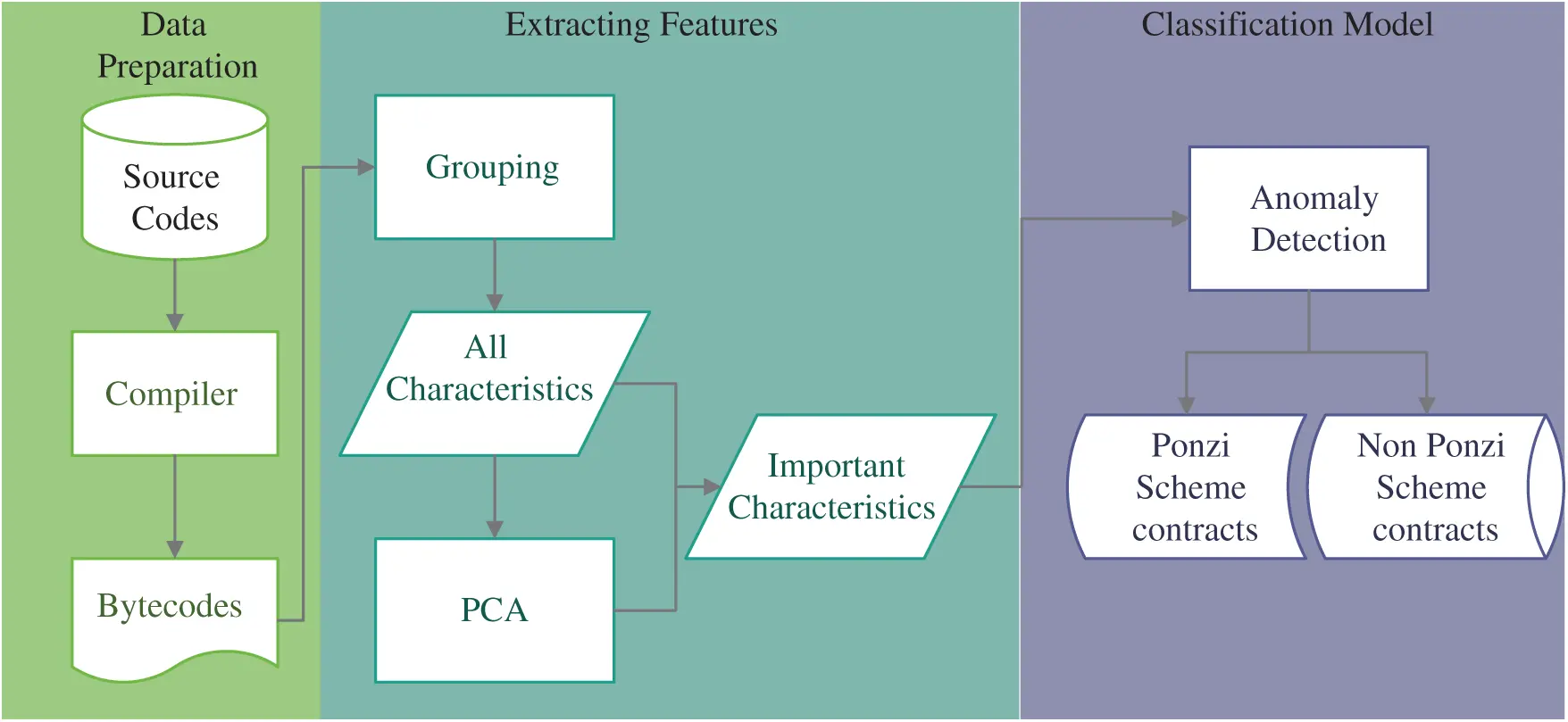Figure 1: Overall framework of the detection model of Ponzi scheme contracts

The main contributions of this paper can be summarized as follows:

1) We innovatively propose to detect Ponzi scheme contracts using the characteristics of bytecodes in smart contracts. First, the bytecodes are transformed into a high-dimensional characteristic matrix. Then, the noise in the characteristic matrix is eliminated using the PCA algorithm. This method improves the generalizability and practicality of the proposed model given that bytecode files are very easy to obtain.

2) We transform the detection problem of the Ponzi scheme contract into an anomaly detection problem by using the characteristics in the bytecodes. This way can significantly improve the accuracy of Ponzi scheme contract detection with only a small number of samples. The F1-score value of Ponzi scheme contract detection in this paper is improved to 88%, which is much better than those of other methods.

The remaining sections of the paper are organized as follows. In Section 2, we introduce the work related to Ponzi scheme contract detection. In Section 3, we elaborate on the proposed detection model of the Ponzi scheme contracts. In Section 4, we perform an experimental evaluation of the proposed model. We conclude and discuss the paper in Section 5.

2  Related Work

This section briefly introduces the differences between non-Ponzi and Ponzi scheme contracts. First, an ordinary smart contract is used as an example to illustrate the steps of executing smart contracts in Ethernet from compilation to deployment. Then, this section analyzes the features of Ponzi schemes contracts in detail. Finally, this section presents the work of other researchers.

2.1 Smart Contract Deployment

Smart contracts in Ethernet are mainly written in the Solidity script language. Thus, this section takes a smart contract written in Solidity as an example. Fig. 2 shows a normal smart contract, which comes from the case in the Solidity language documentation , implementing the voting function.

Line 1 declares the version compiled by Solidity, lines 4–11 define the structural body used in the smart contract, the names of which are “Voter” and “Proposal,” “Voter” includes the information of the elector, and “Proposal” includes the information of the candidate. The values of the variables in the structural body are permanently stored in the storing space of the smart contract. Lines 17–21 are the constructor, which is called only when the smart contract is deployed, and the function is to initialize the “Voter” and “Proposal” structural body. The function in lines 23–26 gives the unvoted voters the right to vote and sets the weight of the vote to 1. The function in lines 28–34 implements the voting function, which is the core logic of this smart contract. Line 29 obtains the information of the elector, and line 30 determines whether this elector has voted; if he or she has already voted, then this elector will fail to vote. Line 31 sets the status of the voter from unvoted to voted. Line 32 indicates that the voter voted for that candidate. Line 33 adds 1 to the number of votes for the candidate who received a vote. Lines 36–43 count the votes of all candidates and find the final winning candidate.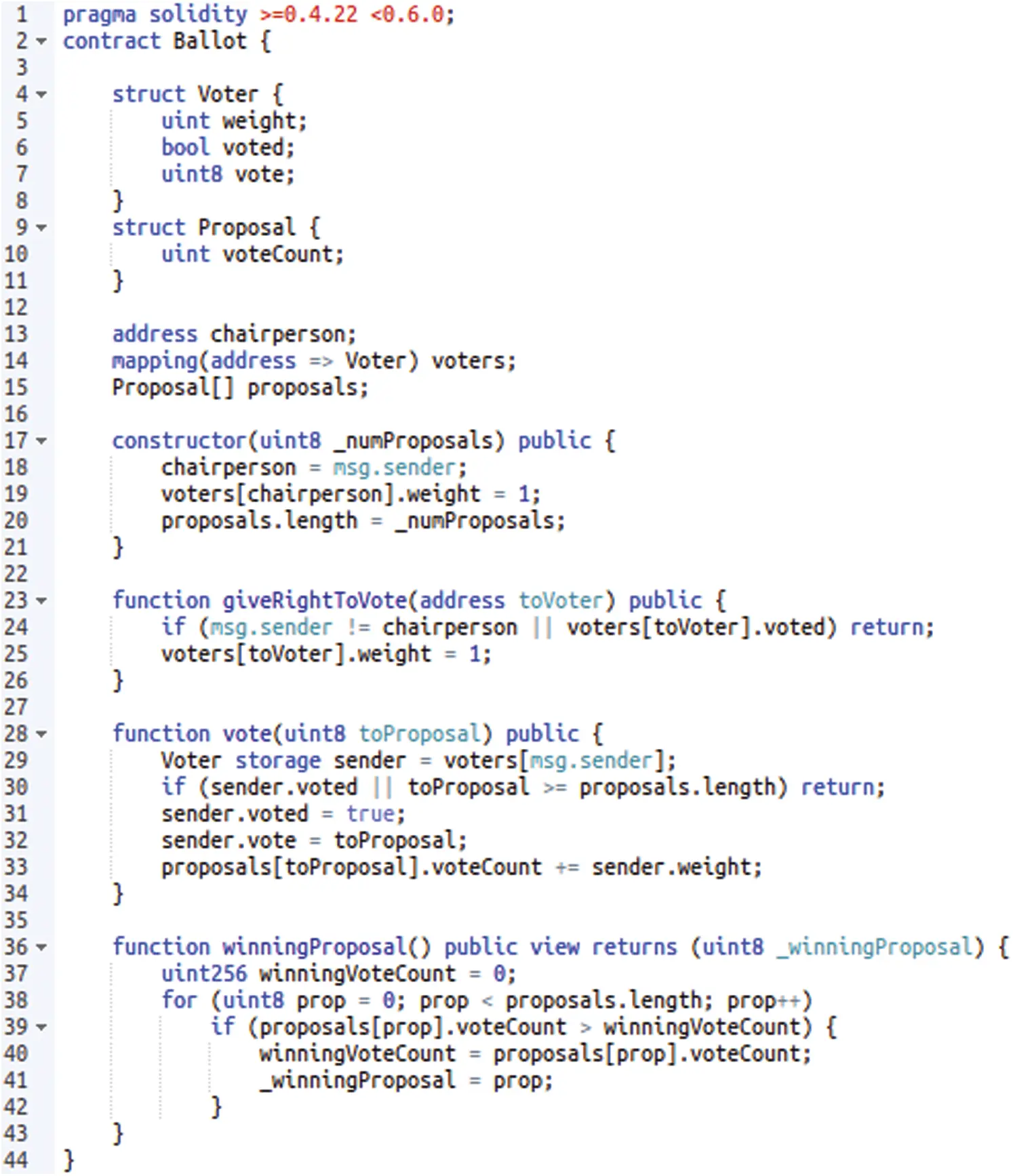Figure 2: Normal smart contracts

The smart contract shown in Fig. 2 is compiled using the Solidity compiler. After successful compilation, the compiler generates two files, the Application Binary Interface (ABI) file and the binary file, which end in “.abi” and “.bin”, respectively. ABI file can be simply understood as the interface description file of the smart contract, which contains the field names, method names, and parameter names, parameter types, in the smart contract. The ABI description with the “vote” function as an example is shown in Fig. 3.Figure 3: ABI description of “vote” function

The binary file of a smart contract contains three parts: deployment code, runtime code, and auxdata. When the EVM executes a smart contract, it will first create the smart contract account and then run the deployment code. After running, it will store the runtime code and auxdata on the blockchain (auxdata is the fingerprint verification of the contract; thus, it will not be executed by EVM). Thereafter, the storage address of both is associated with the smart contract account, and the deployment of the smart contract is completed. The process of deploying smart contracts in Ethernet is shown in Fig. 4. Notably, that only binary codes are required for deploying smart contracts. Thus, the creator can hide the source code of the smart contract. Accordingly, the method of detecting Ponzi scheme contracts based on source code is not feasible. This paper uses runtime code to detect Ponzi scheme contracts given that the runtime code contains the core operational logic of the smart contracts.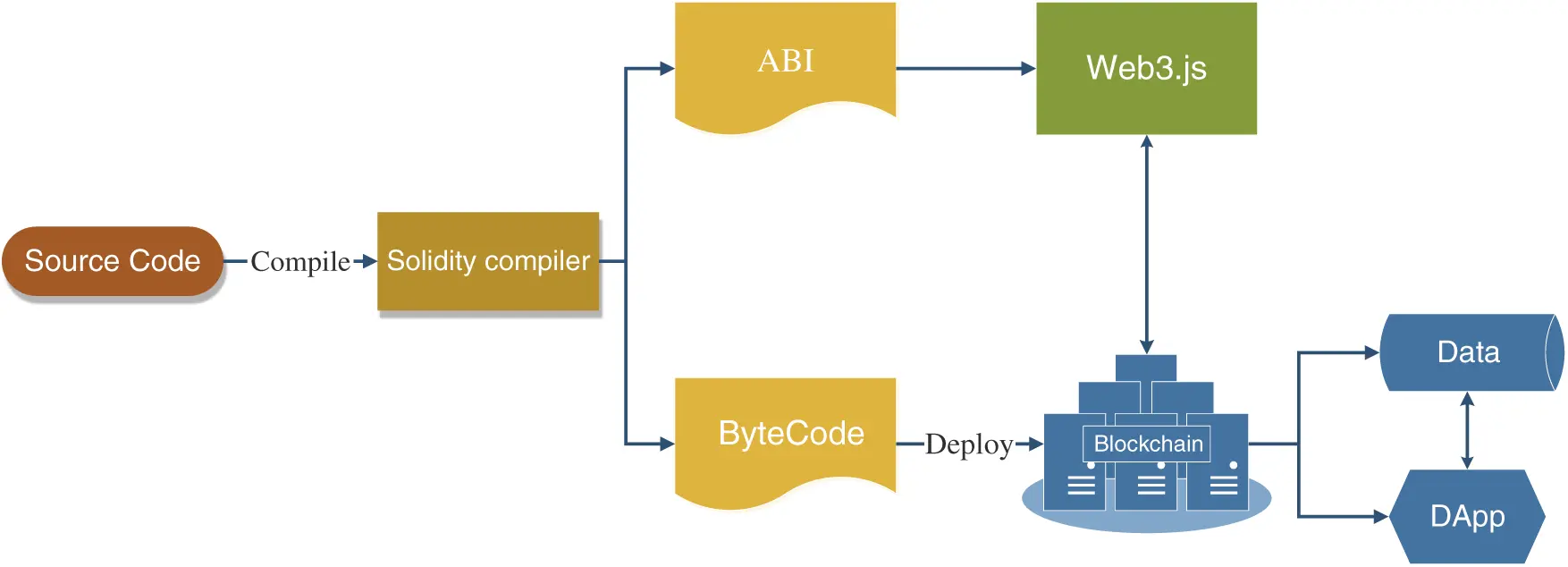Figure 4: Smart contract deployment process

Each byte in the runtime code represents an instruction in the EVM or the number of operations needed to run the instruction. Similar to the JVM, the EVM is a stack-based virtual machine. As a result, all its operations are performed on the stack. The stack in the EVM operates in words, and each word is 32 bytes long, or 256 bits per word, to facilitate cryptographic computation. The EVM stack holds a maximum of 1024 words.

Table 1: Some commonly used opcodes in EVM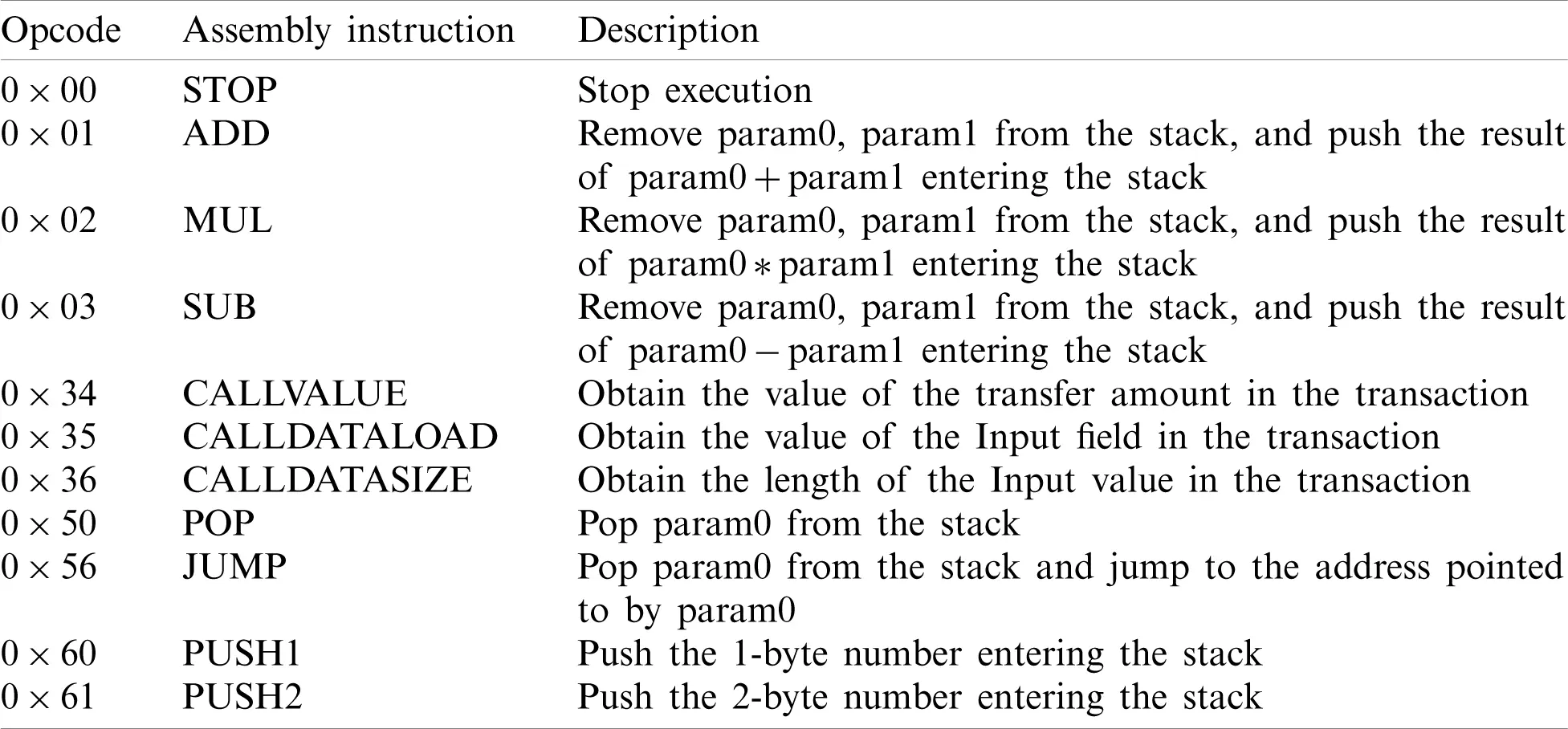Given that EVM limits the length of instructions to one byte, its instruction set can contain up to 256 instructions. Currently, EVM has defined 144 instructions, including jumps, arithmetic operation, comparison, bit operation, and cryptographic computation. Each instruction has a corresponding opcode, and Tab. 1 shows some of the commonly used opcodes in EVM.

2.2 Ponzi Scheme Contract Detection

Vasek et al.  conducted an empirical analysis of Bitcoin fraud for the first time. They concluded four types of scams: Ponzi scheme, mining fraud, fraudulent wallet, and fraudulent exchange. They also pointed out that the fraud amount in Bitcoin fraud reached at least 11 million US dollars. Ngai et al. and Massimo et al. used data mining techniques to identify Ponzi schemes in Bitcoin [22,23]. Massimo et al. analyzed the smart contract scams in Ethereum for the first time. They summarized four salient features in Ponzi scheme contracts through the source code and gave four suggestions . Weili and others extracted the transaction history and opcode features of smart contracts to detect Ponzi scheme contracts. They predicted through this method that more than 500 Ponzi scheme contracts run in Ethereum .

3  Detection Model of Ponzi Scheme Contracts Based on Bytecode

In this paper, we innovatively transform the classification problem of the Ponzi scheme contracts to the anomaly detection problem. Anomaly detection differs from problems and tasks in the conventional paradigm by targeting a small number of unpredictable or uncertain rare events, which have a unique complexity that renders general machine learning and deep learning techniques ineffective [25,26]. Compared with non-Ponzi scheme contracts, Ponzi scheme contracts have very few samples, and their characteristics are very different (see Section 3.1.2 for details). Therefore, this paper converts the classification problem of Ponzi scheme contracts into an anomaly detection problem. A certain degree of rationality exists. The results of the experimental evaluation (Section 4) also verify the rationality of this conversion.

3.1 Data and Feature Extraction

3.1.1 Data

Very few open-source data sets are currently available in the field of Ponzi scheme contract detection. We use the largest known data set of Ponzi scheme contract detection , which is an open-source data set by Weili et al., to ensure the universality and practicability of the proposed model. The data set contains 3780 labeled and 14,000 unlabeled data. A total of 200 pieces of data in the labeled data are marked as Ponzi scheme contracts. These labeled data are obtained after the open-source smart contracts in Etherscan.io are manually checked.

3.1.2 Feature Extraction Based on Bytecode

The developers write the smart contracts and compile them through the Solidity compiler into bytecode that can be run in the EVM. The EVM compiles the bytecode into assembly instructions and then executes the operations in the smart contract through the assembly instructions in the computer. Each calculation step executed by the smart contract will charge a certain amount of fees to avoid network abuse and avoid some of the halting problems caused by Turing completeness. Ethereum calls these fees Gas. Fig. 5 shows the execution process of the smart contract in the EVM.

As concluded from Fig. 5, the bytecode contains all the operations of the smart contract and the data used in the operation. Thus, this paper proposes a detection model of Ponzi scheme contracts based on bytecode. For a start, we use two characters as a group and divide each smart contract into a corresponding characteristic matrix. The advantage of this procedure is that the meaning of the bytecode need not be considered, and various features hidden in the bytecode can be extracted quickly and effectively. After feature extraction, this paper performs standardization operations on these features. We let x denote the value before standardization; μ, σ denote the mean and variance of the value, respectively, and x* denotes the value after standardization. The standardization formula of the features is given as follows:

x*=x-μσ(1)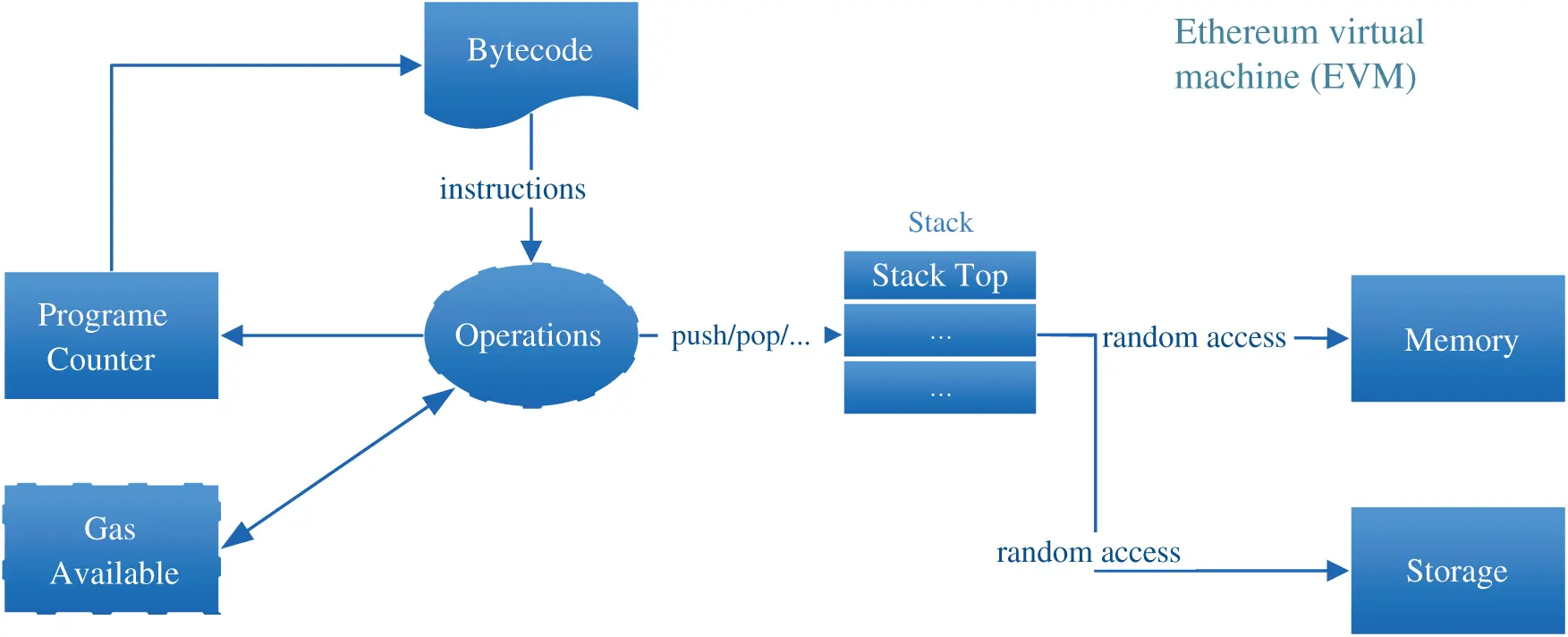Figure 5: The execution process of the smart contract in the EVM

After feature standardization, we find that the characteristic matrix of Ponzi and non-Ponzi scheme contracts are significantly different. The number of Ponzi scheme contracts in the data set used in this paper is 200. Thus, we randomly select 200 samples of non-Ponzi scheme contracts, and Ponzi scheme contract samples are compared. Fig. 6 shows the characteristics of the Ponzi and non-Ponzi scheme contracts. The X-axis is the name of the characteristics, and Y-axis is the mean value of each characteristic. Because of the length, we randomly select 50 features for display.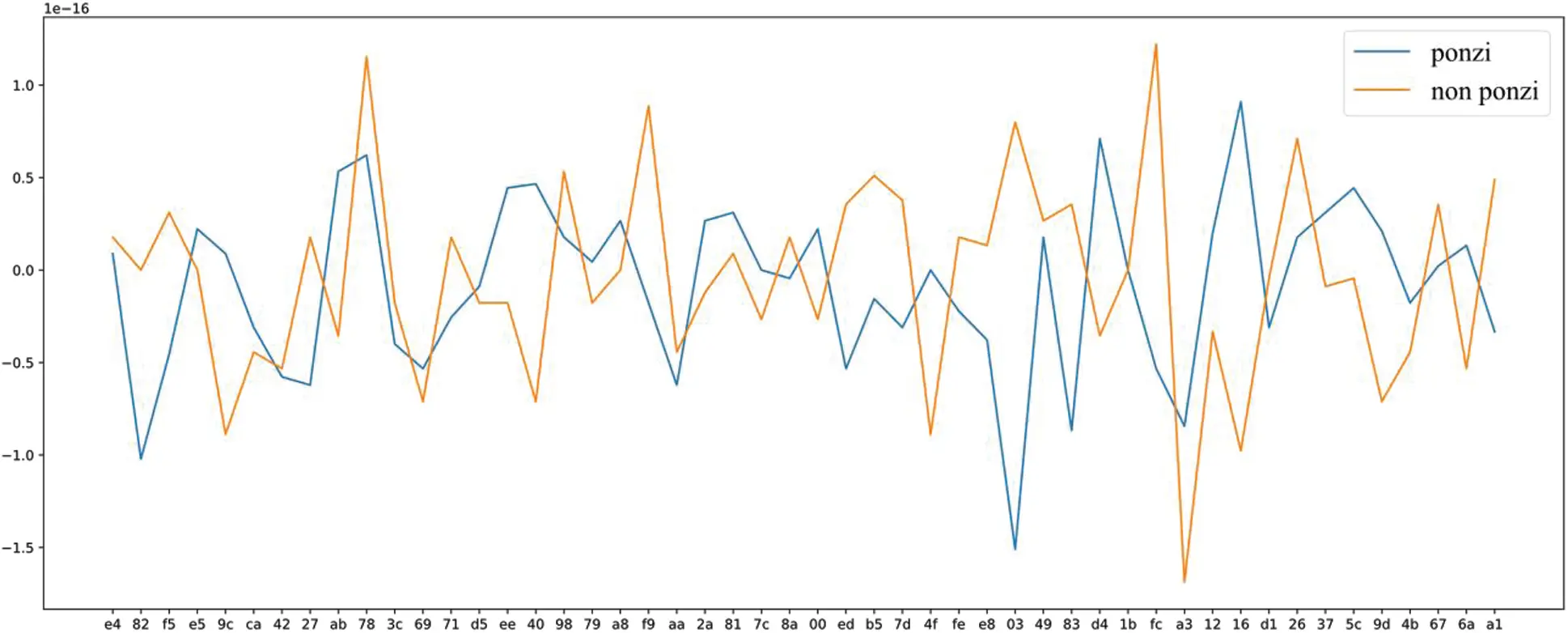Figure 6: Characteristic mean of Ponzi and non-Ponzi scheme contracts (50-dimension)

Although the bytecode contains all the features of the smart contract, it also contains a lot of noise. The PCA algorithm  is used by us to reduce the dimension of the characteristic matrix and eliminate the noise for improving the applicability of the proposed model.

The basic idea of PCA is to map the n-dimensional feature matrix to the k-dimensional characteristic matrix. The new k-dimensional matrix is an orthogonal matrix, also known as the principal component, which is a k-dimensional characteristic reconstructed based on the original n-dimensional characteristic. The PCA calculation process calculates the covariance matrix of the characteristic matrix, then obtains the eigenvalue of the covariance matrix, and finally selects the matrix composed of the eigenvector corresponding to the k features with the largest eigenvalue (that is, the largest variance). In this way, the original space of the matrix can be converted into the new k-dimensional space, and the feature dimension reduction operation can be realized.

Assuming that the elements in sample X are x1,x2,,xn, the elements in sample Y are y1,y2,,yn, x¯,y¯ represent the sample mean of X,Y respectively. The mean formula is given as follows:

x¯=1ninxi(2)

S2 denotes the sample variance, and the formula is shown as follows:

s2=1n-1i=1nxi-x¯(3)

According to the sample mean and variance formula, the covariance formula Cov(X,Y) of X,Y is obtained as follows:

Cov(X,Y)=1n-1i=1n(xi-x¯)(yi-y¯)(4)

Fig. 7 shows the characteristic mean after PCA reduces the original 257-dimensional characteristic to 25-dimensional. As in Fig. 6, we still randomly select 200 samples of non-Ponzi scheme contracts to compare with samples of Ponzi scheme contracts. Notably, the experimental part of this article (Section 4) does not use 25-dimensional characteristics for experiments. In Section 4, we select the best feature dimension according to different algorithms.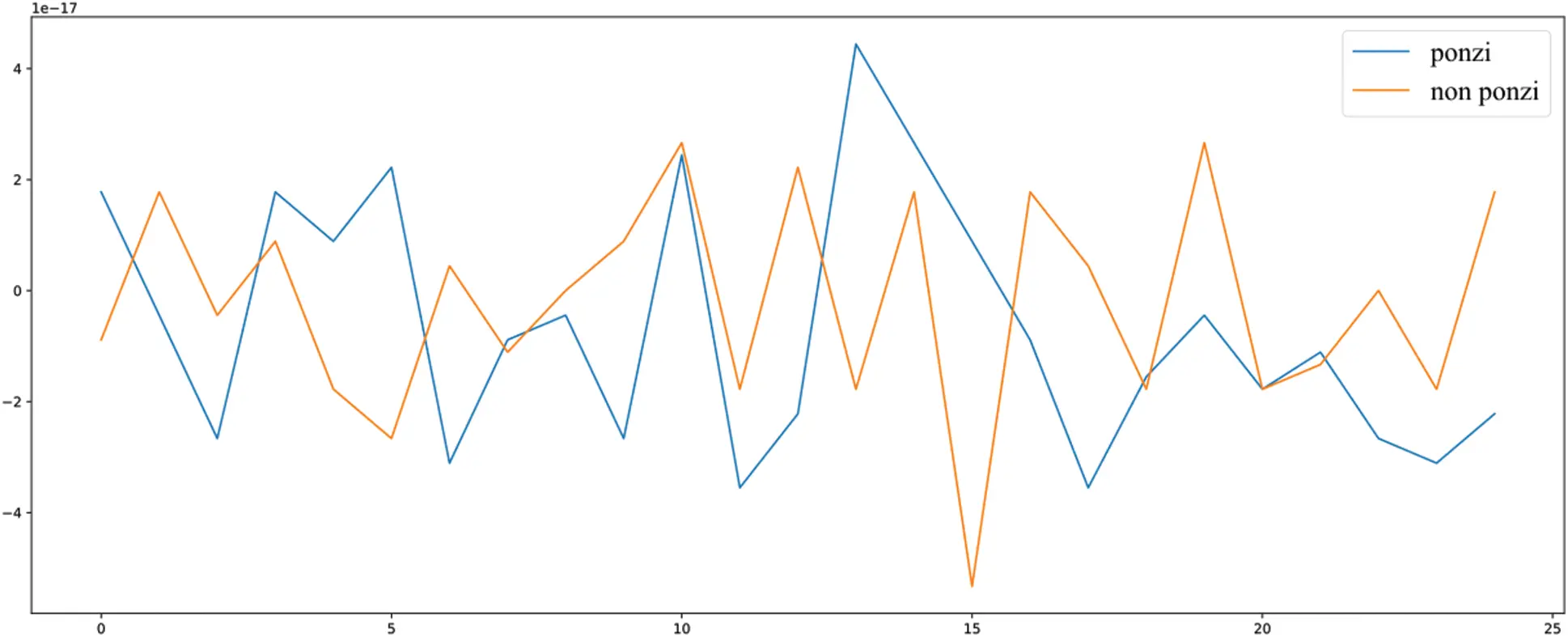Figure 7: 25-dimensional characteristic mean

As shown in Figs. 6 and 7, the bytecode features of Ponzi scheme contracts are extremely different from those of non-Ponzi scheme contracts, which also verifies the rationality of the extracted features in this paper.

3.2 Data Ponzi Scheme Contracts Detection Model

In this paper, the classification problem of Ponzi scheme contracts is transformed into an anomaly detection problem. Then, smart contracts are classified using conventional anomaly detection algorithms. Experiments have proven that these conventional anomaly detection algorithms can also obtain excellent results after the abovementioned feature extraction steps. The anomaly detection algorithms used in this paper are OC-SVM [29,30] and IForest [31,32].

3.2.1 Detection Algorithm of Ponzi Scheme Contracts Based on OC-SVM

OC-SVM is significantly different from the traditional SVM algorithm. The traditional SVM algorithm generally has two types of training data. Its basic model is a linear classifier, which maximizes the interval between different classes in the feature space. However, it can also classify non-linear samples by using the kernel function. The core strategy of the SVM algorithm is to maximize the interval of different types of data, which can be formalized as a problem of solving second-order convex programming. That is, the SVM algorithm is equivalent to the optimization algorithm for solving second-order convex programming.

The SVDD algorithm is widely used in OC-SVM. It can distinguish between target and non-target samples and is usually used in areas such as anomaly detection and fault detection. Its principle is given as follows:

Supposing is a set of single-type training samples XRn*d, n indicates the number of samples, d indicates the feature dimension, and the elements in X are x1,x2,,xn. Supposing that the original space XRd where the data are located, the SVDD algorithm first maps X to the new space through a nonlinear change function ϕ(x)=Zd and Zd indicates the new feature space. Then, it will look for a hypersphere with the smallest volume in the new feature space through the SVDD algorithm. For constructing this smallest hypersphere, SVDD needs to solve the following optimization problems:

mina,R,ξR2+Ci=1nξis.t.ϕ(x)-a2+ξi,ξi0,i=1,2,,n(5)

R indicates the radius of the hypersphere, a indicates the center of the hypersphere, ξ indicates the relaxation factor and C indicates the penalty parameter that weighs the volume of the hypersphere and the misclassification rate. The penalty for misclassification increases when C is large, and the penalty for misclassification rate is reduced when C is small. According to the Lagrange multiplier method, the dual problem of the original problem is

minαi=1nj=1nαiαjK(xi,xj)-i=1nαiK(xi,xj)s.t.0αiC,i=1nαi=1,i=1,2,,n(6)

αi indicates the Lagrangian coefficient corresponding to the sample xi. The calculation formulas for the center a and the radius R of the hypersphere are

a=i=1nαiϕ(x)R=K(xv,xv)-2i=1nαiK(xv,xi)+i=1nj=1nαiαjK(xi,xj)(7)

Among all training samples, the samples with Lagrangian coefficient that satisfies 0αiC are called support vectors, and let SV denote all support vectors in the training sample X. Then, in the formula for calculating R, xvSV, K(xv,xi) indicates the kernel function, which is equivalent to the inner product operation of samples in the feature space, that is

K(xi,xj)=ϕ(xi),ϕ(xj)(8)

Supposing the test sample is xt, the distance d from xt to the center a of the hypersphere is

d=K(xt,xt)-2i=1nαiK(xt,xi)+i=1nj=1nαiαjK(xi,xj)(9)

If dR, then the test sample is inside the hypersphere and is a normal sample; otherwise, it is an abnormal sample.

3.2.2 Figure Detection Algorithm of Ponzi Scheme Contracts Based IForest

IForest is an anomaly detection algorithm based on the Ensemble algorithm family. Thus, it has linear time complexity. IForest is not only fast when processing big data but also has high accuracy. As a result, it has a wide range of applications in the industry. The theoretical basis of IForest has the following two points:

•      The proportion of abnormal data in the total sample size is small.

•      The eigenvalues of abnormal points are very different from those of normal points.

The algorithm idea of IForest is to use a random hyperplane to cut a data space, which can generate two subspaces at a time. Then, a randomly selected hyperplane is used to cut the two subspaces obtained in the first step, and an endless loop is conducted until each subspace contains only one data point. Therefore, those data clusters with high density need to be cut many times to make each point exist in a subspace alone. However, those sparsely distributed points only need to be cut a seldom times to stop in a subspace.

The IForest algorithm is an unsupervised method. First, it randomly selects a feature from the training data set and then randomly selects a split point between the maximum and minimum values of the feature. Points smaller than the split point enter the left branch, and points greater than or equal to the split point enter the right branch. Thereafter, repeat the abovementioned process is repeated until only one sample or similar samples are left. If the tree depth limit is reached in repeating the aforementioned process, then the process will be skipped. Finally, IForest indicates the degree of “isolation” of a sample point by the path length.

Supposing h(x) denotes the number of edges of the binary tree that x passes through in the process of sample point x from the root node to the external node, also known as the path length. Smaller sample path length generally corresponds to a higher degree of “isolation” of this sample. The formula h(x) is given as follows:

h(x)=e+C(n)(10)

The abovementioned formula e indicates the process of x from the root node of the subtree iTree to the leaf node, that is, the number of edges x passes through the iTree. C(n) indicates the average path length of the binary tree constructed using n sample data, and the formula of C(n) is given as follows:

C(n)=2ln(n-1)+γ-2(n-1)n(11)

The aforementioned formula γ indicates the Euler constant. Supposing S(x,n) indicates the abnormal value of x, the formula S(x,n) is shown as follows:

S(x,n)=2-E(h(x))C(n)(12)

E(h(x)) indicates the average path length of x in all iTree. If S(x,n)[0.5,1], then the value S(x,n) is proportional to the probability that x is an abnormal point, that is, the larger the value of S(x,n), which means x is more likely an abnormal point. If S(x,n)[0.5,1], then the value of S(x,n) is inversely proportional to the probability that x is a non-abnormal point, that is, the smaller value of S(x,n), implies x is less likely an abnormal point.

4  Performance Evaluation

In this section, we first describe the experimental environment and experimental indicators. Then, we select four benchmark models for comparative experiments. Finally, we give experimental evaluations of different models.

4.1 Experiment Environment

This paper uses the labeled data set mentioned in Section 3.1 to evaluate our model. This data set has a total of 3780 pieces of data, 200 of which are marked as Ponzi schemes. First, we select 80% of all data as the training set and 20% of the entire data as the test set for the experiment to ensure the accuracy and validity of the experimental results. Then, we select the different proportions of Ponzi and non-Ponzi scheme contracts to conduct experiments for simulating the proportion of Ponzi and non-Ponzi scheme contracts in a real environment. The proportion is set to three gradients, namely PSC: Non_PSC={0.01:0.99,0.05:0.95,0.1:0.9}. We conduct all experiments five times and took an average of five times as the final result.

4.2 Experiment Index

The number of samples of Ponzi and non-Ponzi scheme contract data is quite different. Thus, this paper chooses three metrics, namely, Precision, Recall, and F1-score, to evaluate the performance of the model. The formulas of the three evaluation indicators are given as follows:

Precision=PositivetruePositivetrue+Positiveflase(13)

Recall=PositivetruePositivetrue+Negativeflase(14)

F1-score=2*Precision*RecallPrecision+Recall(15)

4.3 Baseline

This paper selects the following four models as the benchmark model:

•      SVM: Its basic model is to define a linear classifier with the largest interval in the feature space, but it can also classify nonlinear samples by using a kernel function. The core strategy of the SVM algorithm is to maximize the interval of different types of data, which can be formalized as a problem of solving second order convex programming. That is, the SVM algorithm is equivalent to the optimization algorithm for solving second-order convex programming.

•      Random Forest (RF): It is a very representative Bagging integration algorithm. Its basic idea is to randomly select a part of the sample from the overall sample for training, then obtain multiple random results, and finally vote on these results. It uses the voted mean as the final result. The purpose of this way is to avoid poor sample data as much as possible for improving accuracy.

•      Extreme Gradient Boosting (XGBoost) : It is an integrated machine learning algorithm based on decision trees. It uses gradient boost as the framework and uses additive models and forward step-by-step algorithms to achieve the learning optimization process. XGBoost has been applied to many cutting-edge industries because of its excellent performance.

•      Light Gradient Boosting Machine (LightGBM): Similar to XGBoost, it is also a member of the ensemble learning algorithm. However, compared with XGBoost, it does not need to calculate information gain through all samples. It has built-in feature dimensionality reduction technology and thus performs faster and uses lower memory than XGBoost.

4.4 Result Analysis

On the basis of the abovementioned description, Tab. 2 and Fig. 8 show the experimental results of different models. They show the performance evaluation results of the Ponzi scheme contract detection using the aforementioned algorithms. We enumerate the Precision, Recall, and F1-score of different algorithms. For the different parameters of each algorithm, we use the heuristic search to find the best combination of parameters.

Table 2: Experimental results data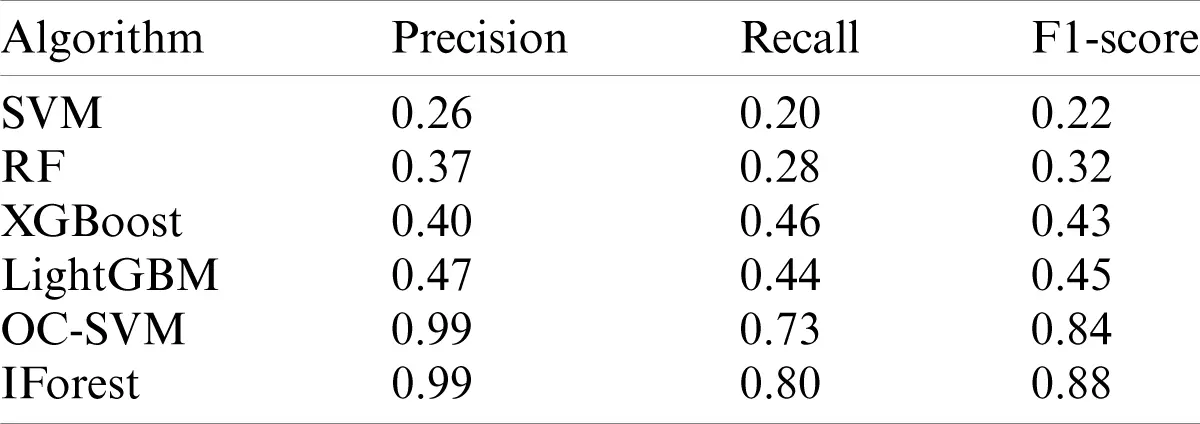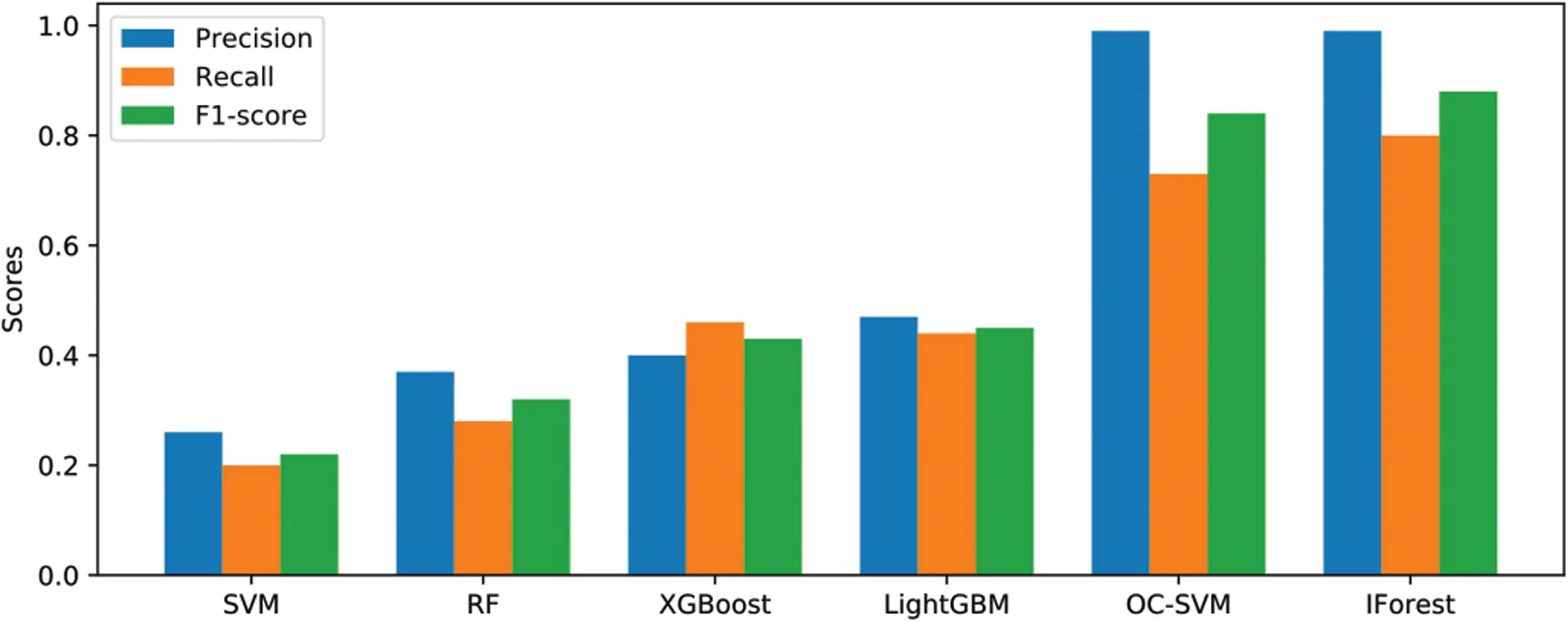Figure 8: Experimental results

The operating system of this experiment is Ubuntu 18.04, Python version: 3.7.4, sklearn library version is 0.22.1, CPU model is Inter Core i5-8500, memory size is 8 G.

For the SVM algorithm, we use Gaussian kernel as the kernel function, and optimizes the kernel coefficient gamma={0.01,0.02,0.03,,0.1} and the sample class_weight={10,11,12,,20}. In the test, the best combination is gamma=0.08, class_weight=14. For RF, we adopt a similar strategy to search for n_estimators={1,2,3,,100} heuristically, and obtain n_estimators=83 as the best value. For XGBoost, we optimize eta={0.1,0.15,0.3}, max_depth,={4,5,6,7}, scale_pos_weight={10,11,12,,20} to obtain the best the combination is eta=0.15, max_depth,=6, scale_pos_weight=20. For LightGBM, we optimize the combination of n_estimators={100,110,120,,200}, learning_rate={0.01,0.15,0.3}, scale_pos_weight={5,6,7,,15}, and obtain the best combination as n_estimators=150, learning_rate=0.01, scale_pos_weight=10. The best combination for IForest algorithm is n_estimators=150, max_samples=50. The best combination for OC-SVM is nu=0.01, kernel=rbf, gamma=0.02. For the algorithm parameters not mentioned, we all use the default parameters of the algorithm.

Tab. 2 and Fig. 8 show that the values of Precision, Recall, and F1-score of the IForest and OC-SVM models are far superior to those of other models. We simulate the performance difference between IForest and OC-SVM in the real environment to further explore their best performance by changing the proportion of Ponzi and non-Ponzi scheme contracts in the sample. The proportion of Ponzi and non-Ponzi scheme contracts set in this paper is PSC:Non_PSC={0.01:0.99,0.05:0.95,0.1:0.9}. The results are shown in Tab. 3 and Fig. 9.

Table 3: Data of different proportions of Ponzi and non-Ponzi scheme contracts on the model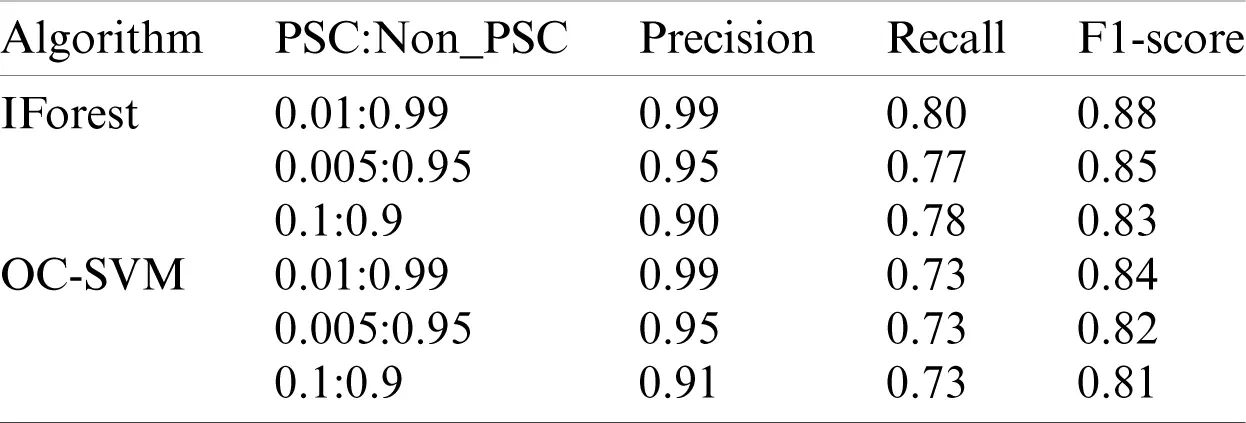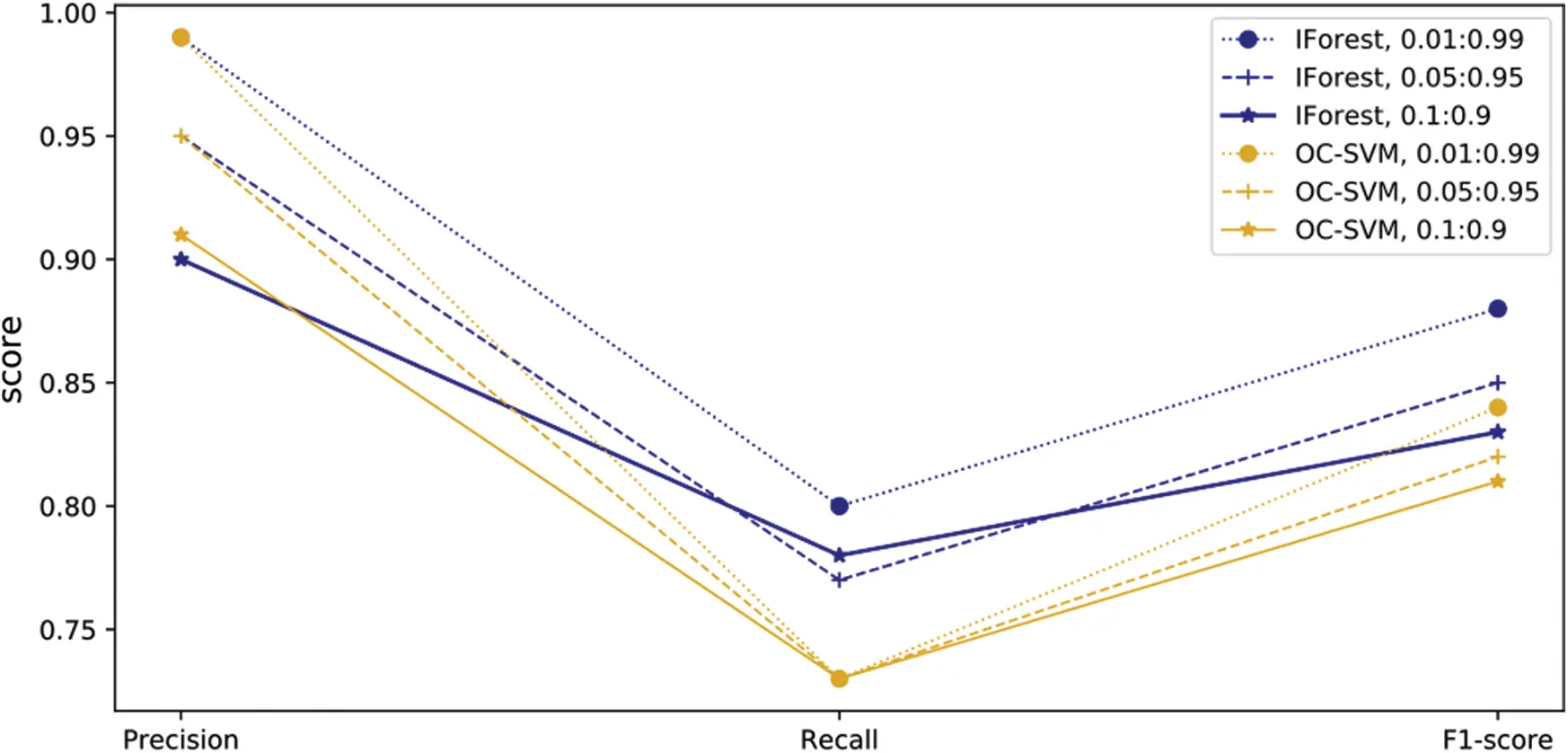Figure 9: Different proportions of performance of Ponzi and non-Ponzi scheme contracts

From Tab. 3 and Fig. 9, the following conclusions can be made.

1) The performance of the IForest model is much better than that of the OC-SVM model.

2) The performance of the IForest and OC-SVM models gradually decreases as the proportion of Ponzi scheme contracts increases. The reason is that the proportion of Ponzi and non-Ponzi scheme contracts increasingly does not meet the definition of anomaly detection problems as the proportion of Pozi scheme contracts increases. As a result, so the performance of IForest and OC-SVM gradually declines.

3) The Precision of the IForest model has reached 0.99, and F1-score has also reached 0.88. Thus, the proposed method is effective for detecting Ponzi schemes contracts, and its performance is far better than those of other models.

5  Conclusion and Future Work

The Ponzi scheme contracts on the blockchain will cause the asset loss of the user. Thus, detecting the Ponzi schemes contracts is a vital task. This paper proposes a bytecode-based detection model of Ponzi scheme contracts, innovatively transforms the classification of Ponzi scheme contracts into anomaly detection problems, and implements its prototype. First, we extract all the features in the bytecode and convert the bytecode into a characteristic matrix. Then, the PCA method is used to reduce the dimensionality of the characteristic matrix for eliminating the noise in the characteristic matrix and improving the accuracy of detection. Finally, we use the proposed bytecode-based anomaly detection algorithm to identify Ponzi scheme contracts. The experimental results prove that the method proposed is far superior to other methods.

In the future, we will continue to research from two aspects. First, we will continue to dig deeper into the features contained in the bytecode to further improve the detection of Ponzi scheme contracts. Second, we hope to build a real-time detection platform in Ethereum to purify the network environment.

Funding Statement: This work was supported by the Scientific and Technological Project of Henan Province (Grant No. 202102310340), Foundation of University Young Key Teacher of Henan Province (Grant Nos. 2019GGJS040, 2020GGJS027), Key Scientific Research Projects of Colleges and Universities in Henan Province (Grant No. 21A110005), and National Natual Science Foundation of China (61701170).

Conflicts of Interest: The authors declare that they have no conflicts of interest to report regarding the present study.

## References

1. Nakamoto, S. (2008). Bitcoin: A peer-to-peer electronic cash system. https://bitcoin.org/bitcoin.pdf.
2. Wang, Y., Tian, Z., Sun, Y., Du, X., & Guizani, N. (2020). Locjury: An IBN-based location privacy preserving scheme for IOCV. IEEE Transactions on Intelligent Transportation Systems, 21(1), 30-47. [Google Scholar] [CrossRef]
3. Tian, Z., Gao, X., Su, S., Qiu, J., & Du, X. (2019). Evaluating reputation management schemes of internet of vehicles based on evolutionary game theory. IEEE Transactions on Vehicular Technology, 68(6), 5971-5980. [Google Scholar] [CrossRef]
4. Qiu, J., Du, L., Zhang, D., Su, S., & Tian, Z. (2020). Nei-TTE: Intelligent traffic time estimation based on fine-grained time derivation of road segments for smart city. IEEE Transactions on Industrial Informatics, 16(4), 2659-2666. [Google Scholar] [CrossRef]
5. Wrightw, C., Serguieva, A. (2017). Sustainable blockchain-enabled services: smart contracts. IEEE International Conference on Big Data, pp. 4255–4264. Boston, MA, DOI 10.1109/BigData.2017.8258452. [CrossRef]
6. Tian, Z., Li, M., Qiu, M., Sun, Y., & Su, S. (2019). Block-DEF: A secure digital evidence framework using blockchain. Information Sciences, 491(3), 151-165. [Google Scholar] [CrossRef]
7. Gu, J., Sun, B., Du, X., Wang, J., & Zhuang, Y. (2018). Consortium blockchain-based malware detection in mobile devices. IEEE Access, 6(1), 12118-12128. [Google Scholar] [CrossRef]
8. Securities, U., Commission, E. (2017). Report of investigation pursuant to section 21(a) of the securities exchange act of 1934: The DAO. http://www.virtualschool.edu/mon/Economics/ SmartContracts.html.
9. Palladino, S. (2017). The parity wallet hack explained. Openzeppelin. https://blog.zeppelin.solutions/on-the-parity-wallet-multisighack-405a%8c12e8f7/.
10. Wikipedia (2017). Ponzi scheme. Eikipedia. https://en.wikipedia.org/wiki/Ponzi_scheme.
11. Higgins, S. (2015). SEC seizes assets from alleged altcoin pyramid scheme. Coindesk. https://www.coi7ndesk.com/sec-seizesalleged-altcoin-pyramid-scheme.
12. Keirns, G. (2017). Gemcoin ponzi scheme operator hit with \$74 million judgment. Bitcoinwiki. https://bitcoinwiki.co/gemcoinponzi-scheme-operator-hit-with-74-million-judgment/.
13. Chen, W., Zheng, Z., Ngai, E. C. H., Zheng, P., & Zhou, Y. (2019). Exploiting blockchain data to detect smart ponzi schemes on ethereum. IEEE Access, 7, 37575-37586. [Google Scholar] [CrossRef]
14. Qiu, J., Tian, Z., Du, C., Zuo, Q., & Su, S. (2020). A survey on access control in the age of Internet of Things. IEEE Internet of Things Journal, 7(6), 4682-4696. [Google Scholar] [CrossRef]
15. Shafiq, M., Tian, Z., Bashir, A. K., Du, X., & Guizani, M. (2020). Corrauc: A malicious bot-iot traffic detection method in iot network using machine learning techniques. IEEE Internet of Things Journal, 8(5), 3242-3254. [Google Scholar] [CrossRef]
16. Li, M., Sun, Y., Lu, H., Maharjan, S., & Tian, Z. (2019). Deep reinforcement learning for partially observable data poisoning attack in crowdsensing systems. IEEE Internet of Things Journal, 7(7), 6266-6278. [Google Scholar] [CrossRef]
17. Morris, D. Z. (2017). The rise of cryptocurrency ponzi schemes. https://www.theatlantic.com/technology/archive/2017/05/cryptocurrency-ponzi-schemes/528624/.
18. Luo, C., Tan, Z., Min, G., Gan, J., Shi, W. et al. (2020). A novel web attack detection system for Internet of Things via ensemble classification. IEEE Transactions on Industrial Informatics, 1. DOI 10.1109/TII.2020.3038761. [CrossRef]
19. Sun, Y., Tian, Z., Li, M., Su, S., Du, X. et al. (2020). Honeypot identification in softwarized industrial cyber-physical systems. IEEE Transactions on Industrial Informatics, 1. Early Access, DOI 10.1109/TII.2020.3044576. [CrossRef]
20. Swan, M. (2015). Blockchain: Blueprint for a new economy, pp. 1362–1364. England: O’Reilly, pp. 1362-1364.
21. Vasek, M., Moore, T. (2015). There’s no free lunch, even using bitcoin: Tracking the popularity and profits of virtual currency scams. In: Böhme, R., Okamoto, T. (Eds.), Financial cryptography and data security. Lecture notes in computer science, pp. 44–61. Berlin, Heidelberg: Springer.
22. Ngai, E., W, T., Hu, Y., Wong, Y. H., & Chen, Y. J. (2010). The application of data mining techniques in financial fraud detection: A classification framework and an academic review of literature. Decision Support Systems, 50(3), 559-569. [Google Scholar] [CrossRef]
23. Bartoletti, M., Pes, B., Serusi, S. (2018). Data mining for detecting bitcoin ponzi schemes. 2018 Crypto Valley Conference on Blockchain Technology, pp. 75–84. Zug, Switzerland, DOI 10.1109/CVCBT.2018.00014. [CrossRef]
24. Bartoletti, M., Carta, S., Cimoli, T., & Saia, R. (2019). Dissecting ponzi schemes on ethereum: Identification, analysis, and impact. Future Generation Computer Systems, 102(3–4), 259-277. [Google Scholar] [CrossRef]
25. Pang, Y., Peng, L. Z., Chen, Z. X., Yang, B., & Zhang, H. L. (2019). Imbalanced learning based on adaptive weighting and gaussian function synthesizing with an application on android malware detection. Information Sciences, 484(1), 95-112. [Google Scholar] [CrossRef]
26. Mi, Y., Liu, W., Shi, Y., Li, J. (2020). Semi-supervised concept learning by concept-cognitive learning and concept space. IEEE Transactions on Knowledge and Data Engineering, 1. DOI 10.1109/TKDE.2020.3010918. [CrossRef]
27. Chen, W. L., Zheng, Z. B., Cui, J. H., Ngai, E., Zheng, P. et al. (2018). Detecting ponzi schemes on ethereum: Towards healthier blockchain technology. Proceedings of the 2018 World Wide Web, pp. 1409–1418. Geneva, Switzerland, DOI 10.1145/3178876.3186046. [CrossRef]
28. Turk, M., & Pentland, A. (1991). Eigenface for recognition. Journal of Cognitive Neuroscience, 3(1), 71-86. [Google Scholar] [CrossRef]
29. Schölkopf, B., Williamson, R. C., Smola, A. J., Shawe-Taylor, J., Platt, J. C. (1999). Support vector method for novelty detection. Advances in Neural Information Processing Systems, 12, pp. 582–588.
30. David, M. T., & Duin, R. P. (2004). Support vector data description. Machine Learning, 54(1), 45-66. [Google Scholar] [CrossRef]
31. Liu, F. T., Ting, K. M., Zhou, Z. H. (2008). Isolation forest. 2008 Eighth IEEE International Conference on Data Mining, pp. 413–422. Pisa, Italy, DOI 10.1109/ICDM.2008.17. [CrossRef]
32. Liu, F. T., Ting, K. M., & Zhou, Z. H. (2012). Isolation-based anomaly detection. ACM Transactions on Knowledge Discovery from Data, 6(1), 1-39. [Google Scholar] [CrossRef]
33. Chen, T. Q., Guestrin, C. (2016). XGBoost: A scalable tree boosting system. Proceedings of the 22nd ACM SIGKDD International Conference on Knowledge Discovery and Data Mining, pp. 785–794. New York, NY, USA, DOI 10.1145/2939672.2939785. [CrossRef]This work is licensed under a Creative Commons Attribution 4.0 International License, which permits unrestricted use, distribution, and reproduction in any medium, provided the original work is properly cited.